# Formulas of reduced multiplication

Formulas of reduced multiplication formulas to multiply polynomials, are used for the decomposition of these polynomials into factors, simplify expressions, and the construction of the polynomials to the standard view. Formulas of reduced multiplication to prove directly by opening the brackets and construction of these terms.

## The formula of squares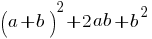the square of the sum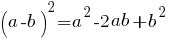the squared differencethe difference of squares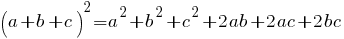## Formula cubic metersthe cube of the sum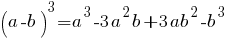cube of a differencethe sum of cubes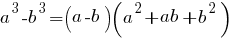difference of cubes

## Formulas of reduced multiplication to the fourth power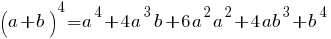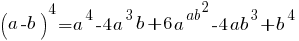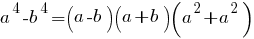The square of the sum of two numbers is equal to the square of the first number plus twice the product of the first number by the second, plus the square of the second number.

The square of the sumThe difference between the squares of two numbers is equal to the product of the sum of these numbers is to their difference.

The difference of squares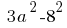The cube is the sum of two numbers is equal to waste the first day plus triple the square of the product of the first number by the second, tripled plus the product of the first number by the square of the second plus the cube of the second number.

The cube of the sumCube of the difference is equal to the boiler of the first number minus tripled the product of the square of the first number by the second, tripled plus the product of the first number by the square of the second minus the cube of the second number.

Cube of a difference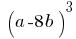The sum of the cubes of two numbers is equal to the product of the sum of these numbers on the incomplete square of the difference of these numbers.

The sum of cubes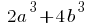The difference between the cubes of two numbers is equal to the product of the difference of the numbers on the incomplete square of the sum of these numbers.

Difference of cubes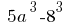Versions in other languages:
Share with friends: